e-ISSN:2320-1215 p-ISSN: 2322-0112

All submissions of the EM system will be redirected to Online Manuscript Submission System. Authors are requested to submit articles directly to Online Manuscript Submission System of respective journal.

# Mechanical and Thermal Kinetic Parameters of Metformin Hydrochloride Crystals.

Ramukutty S, Jeyasudha R and Ramachandran E*

Department of Physics, Thiruvalluvar College, Papanasam – 627425, Tamilnadu. India.

*Corresponding Author:
Department of Physics, Thiruvalluvar College, Papanasam – 627425, Tamilnadu. India.

Received date: 12/01/2014 Accepted date: 13/03/2014 Revised date: 21/02/2014

Visit for more related articles at Research & Reviews in Pharmacy and Pharmaceutical Sciences

## Abstract

Metformin hydrochloride single crystals of large size: 15.5 × 1 × 1.5 mm3 were grown in aqueous solution by solvent evaporation method. Crystal structure of metformin hydrochloride was confirmed using single crystal X–ray diffraction analysis. Work-hardening coefficient and Newtonian resistance pressure of the crystal has been estimated using Vickers hardness measurement. Thermal analysis was performed under isothermal condition in the temperature range of 25–700°C. Coats–Redfern relation was used to evaluate the thermal data for the determination of kinetic and thermodynamic parameters. The Arrhenius equation of metformin hydrochloride is k = 0.60 x 1010 e –137473/RT mol–1s–1.

#### Keywords

Metformin hydrochloride, XRD, Hardness, Thermogravimetric analysis.

#### Introduction

Metformin hydrochloride (1,1–Dimethylimidodicarbonimidic diamide hydrochloride) is an antihyperglycemic drug used in the treatment of type 2 diabetes. Particularly, it is used for the obese people those with normal kidney function and with overweight. Many works related to pharmaceutical properties of metformin hydrochloride were reported in literature [1,2,3], but the physical and thermal properties were less studied. Thermogravimetric analysis (TGA) gives information about the thermal stability, oxidative stability and decomposition kinetics of materials. TGA was applied in the pharmaceutical industry to reveal physicochemical properties of drug, polymorphism, purity and formulation compatibility. Thermal analysis of gypsum crystals  and some drug crystals [5,6,7] were evaluated by the authors.

Presently, single crystals of metformin hydrochloride were grown by slow solvent evaporation method. The grown crystal was confirmed using single crystal X–ray diffraction analysis. Kinetic parameters were evaluated from TG data using Coats and Redfern method. Thermal stability and thermodynamic parameters were also determined.

#### Experimental

Glycomet 500 SR drugs were purchased from USV Ltd, India. Aqueous solution MH of concentration 0.75 M was prepared using the double distilled UV treated water. Glass dishes (50 mm diameter) were used as crystal growth vessels. Crystallization was allowed to take place in a crystal growth chamber having constant ambient temperature ≈27°C and photo proof environment. Transparent, well defined shaped crystals of size: 15.5 × 1 × 1.5 mm3 (Fig. 1) were crystallized within few days.

The grown crystals were subjected to X–ray diffraction studies using Enraf–Nonius CAD–4 Diffractometer, using MoKα (λ= 0.71073 Å). Cell parameters were obtained from least–squares refinement of the setting angles of 79 reflections.

The microhardness of the grown crystals was measured using a Shimadzu Microhardness Tester (Model No. HMV2T) with a diamond indenter. Well polished crystal was mounted on a platform of the microhardness tester and different loads were applied over a fixed interval of time. The indentation time was fixed as 15s. Diagonal lengths of the indentation (d) were measured for various loads (P) in low load region (LLR). Vickers hardness number was determined using the expression (1) .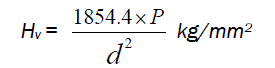(1)

Simultaneous thermogravimetric analysis (TGA) and differential thermal analysis (DTA) were carried out for the powdered sample of as grown metformin hydrochloride crystals in the temperature range 25°C to 700°C with a constant heating rate of 15°C/min under nitrogen atmosphere using Perkin Elmer, Diamond thermal analyzer. The crucible used was made of alumina which served as a reference for the sample. TGA curve of metformin hydrochloride is shown in Fig. 2.

#### X–ray diffraction analysis

The lattice parameters are: a = 7.99(7) Å, b = 14.01(23) Å, c = 7.99(8) Å, β=114.85(16)° and the space group is P21/a. These values agree well with the values reported value . These values confirm the identity of metformin hydrochloride.

#### Hardness analysis

Figures 3a and 3b show the variation of log d versus log P and of P versus d2 respectively. It is found that the microhardnesss increases with increase of load. The expression (2) relating load and diagonal length of the indenter is given by Meyer’s law as,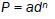(2)

where, P is the load, n is the work hardening coefficient and a is material constant. According to Hays and Kendall  when a load is applied to a material, P is partially affected by a small resistance pressure. Based on this hypothesis, eqn. (2) was modified as,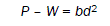(3)

where, W is the Newtonian resistance pressure and b is a constant.

The plot of log d versus log P gives a straight line (Fig. 3a) and the work hardening is calculated as 3.39. In microhardness studies, the hardness number should increase with the increase of load, if n > 2 and decrease if n < 2. Onitsch  has pointed out that n lies between 1.0 and 1.6 for hard materials and more than 1.6 for soft materials. According to this criterion, metformin hydrochloride is considered as a soft material and the material constant is determined as 2.950 x 10-4 g/μm2. The plot between P and d2 (Fig. 3b) is almost linear and the Newtonian resistance pressure and the constant (b) are estimated as –37.747 g and 0.0743 g/μm2 respectively. Newtonian resistance pressure is negative in LLR .

The elastic stiffness constant (C11) for different loads was calculated using Wooster’s empirical formula C11 = Hv7/4and are given in Table 1.

#### Thermal analysis

In the TGA/DTA curve of metformin hydrochloride the first endothermic peak at 229.89°C indicates the melting point of metformin hydrochloride . The mass loss happened in five stages. The first mass loss of 10.28% at 239.9°C is attributed to the release of NH3 molecule from the title compound 1,1-Dimethylbiguanide hydrochloride and an exothermic trend affirms this dissociation. An unstable intermediate compound C8H19N9.2HCl and an end product 1,1-dimethyl-2-cyanoguanidine hydrochloride may result in this process. The formation of this compound may be justified by the mass loss 5.1% equivalent to half molecule of NH3 evolved due to dimer formation. Further heating results in a loss of 22.02% with the elimination of HCl molecule at 275.3 °C from the compound. The liberation of C2H4 molecule contributes a mass loss of 16.94% at 304.9°C and cyanoguanidine (C2H4N4) may be formed. This compound decompose to become dicyanamide (C2HN3) and NH3 resulting with a mass loss of 10.28% at 323.8°C. Further heating results in a mass loss 16.32% (HCN) and 16.91% (N2) at 585.4°C and leaves carbon as residue.

#### Kinetic parameters

Two different equations (4 & 5) are applied to evaluate the kinetic parameters from the TG curve of Fig. 2. Coats and Redfern  derived the following equation to determine the values of activation energy (E), frequency factor (A) and order of the reaction (n).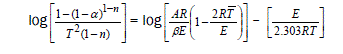(4)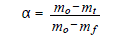(5)

where α – fraction of the substance decomposed at time t, n – order of the reaction, A – frequency factor, E – activation energy, R – gas constant and β – heating rate.

To determine the value of activation energy and order of the reaction, the plot of left hand side of Equation (1) versus 1/T is drawn for different values of n and the best linear plot gives the correct value of n. This equation is valid for all values except n = 1. The best linear plot is obtained for n = 4.5 and is decipted in Fig. 4. Activation energy and frequency factor were determined from the slope and intercept of the linear fit. The calculated values of activation energy and frequency factor are 137.473 kJ/mol and 0.62 x 1010s–1 respectively. The Arrhenius equation for metformin hydrochloride is k = 0.62 x 1010 e –137473/RT mol–1 s–1.

#### Thermodynamic parameters

The Thermodynamic parameters of metformin hydrochloride: entropy of activation (ΔS), enthalpy of activation (ΔH) and Gibbs free energy (ΔG) were calculated using equations 6–8.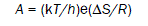(6)

where h and k are Planck’s constant and Boltzmann constant respectively.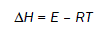(7)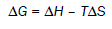(8)

The values of ΔS, ΔH and ΔG are –62.49 J/Kmol, 132.89 kJ/mol and 167.31 kJ/mol respectively. Positive value of ΔG shows that the decomposition reaction of metformin hydrochloride is non–spontaneous.

#### Conclusions

Single crystals of metformin hydrochloride were crystallized by slow solvent evaporation method. Monoclinic form of the crystal was confirmed using single crystal X–ray diffraction analysis. Vickers hardness test revealed that the material belong to soft category. Elastic stiffness and Newtonian resistance pressure have been evaluated. Coats and Redfern relation was applied to determine the kinetic parameters and Arrhenius equation. Thermodynamic parameters showed that the decomposition reaction is non–spontaneous.

#### Acknowledgement

The authors thank the University Grants Commission, Government of India, for providing Major Research Project; the Secretary of Thiruvalluvar College, Papanasam and Dr. K. Ravikumar, Dr. B. Sridhar, Laboratory of X–ray Crystallography, IICT, Hyderabad.